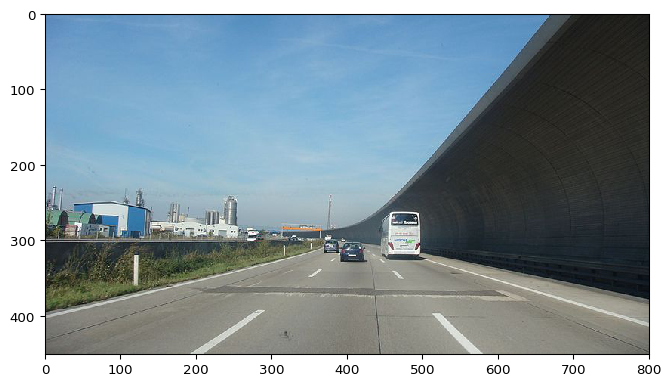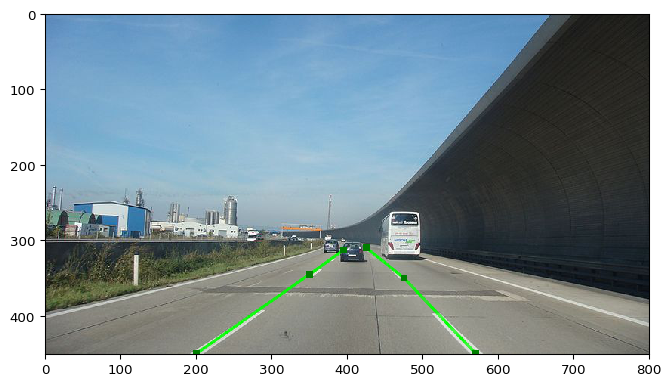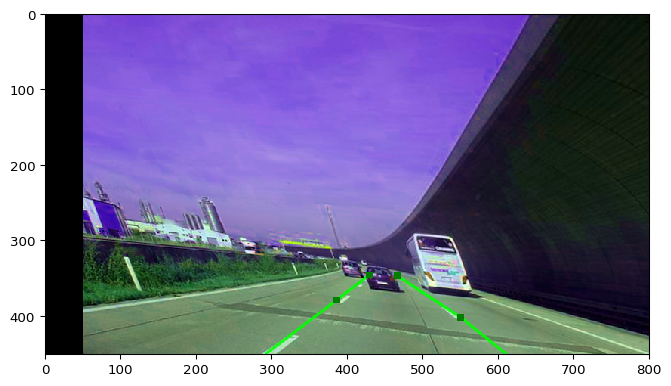# Augment Line Strings¶

Note: The following document is very similar to the one for polygon augmentation. If you have already read that one, you can probably just quickly scroll through this one.

imgaug has native support for line string augmentation (currently in Beta state). A line string is a collection of many single line segments, with each having a start and end point. Line strings are -- in contrast to polygons -- not closed, i.e. no connection will be drawn or assumed between the first and last point of the whole line string.

## API¶

The following classes are relevant for line string augmentation:

### API: LineString¶

imgaug.augmentables.lines.LineString(coords, [label]): Container for a single line string. The coords are the points that (when connected) form the line string. They are provided as (sub-)pixel absolute coordinates. E.g. LineString([(0, 0), (10, 0), (10, 10)]) creates a line string with a 90° angle at x=10, y=0.

• Properties offered by LineString are: .coords (all points), .label (the line string's class label, may be None), .xx (x-coordinates of all points), .yy (analogous), .xx_int (.xx rounded and cast to integers), .yy_int (analogous), .height (height of the line string), .width (analogous) and .length (euclidean distance between each pair of consecutive points).
• Methods offered by LineString are:
• get_pointwise_inside_image_mask(image): Generate for each point whether it is inside the provided image shape (True/False).
• compute_neighbour_distances(): Generate a list of euclidean distances between consecutive points in coords.
• compute_pointwise_distances(other, default=None): Compute the distance from each point in coords to geometry other (e.g. another line string).
• compute_distance(other, default=None): Compute the minimum distance from any location on the line string to other.
• contains(other, max_distance=1e-4): Return whether other has a distance to the line string of less than max_distance.
• project(from_shape, to_shape): Project the line string from image shape from_shape onto image shape to_shape, i.e. change the point coordinates. This is useful when resizing images.
• is_fully_within_image(image, default=False): Estimate whether no part of the line string is outside of the given image plane.
• is_partly_within_image(image, default=False): Estimate whether any part of the line string is inside of the image plane.
• is_out_of_image(image, fully=True, partly=False, default=False): Estimate whether the line string is at least partly or fully outside of the image plane.
• clip_out_of_image(image): Remove all parts of the line string that are outside of the image plane. This can split line segment, creating new coordinates on the image edges. This also returns a list of 0 to N new line strings.
• find_intersections_with(other): Returns intersections with another object (one list of intersections per line segment).
• shift(x=0, y=0): Move the line string along the x/y-axis.
• draw_mask(image_shape, size_lines=1, size_points=0, raise_if_out_of_image=False): Convert the line string to a binary mask with shape image_shape. An area around the line string of size size_lines will be True in the mask.
• draw_lines_heatmap_array(image_shape, alpha=1.0, size=1, antialiased=True, raise_if_out_of_image=False): Convert the line string to a heatmap array (float array, value range [0.0, 1.0]). This draws only the line segments of the line string, not the points.
• draw_points_heatmap_array(image_shape, alpha=1.0, size=1, raise_if_out_of_image=False): Convert the line string to a heatmap array (float array, value range [0.0, 1.0]). This draws only the point of the line string, not the line(s).
• draw_heatmap_array(image_shape, alpha_lines=1.0, alpha_points=1.0, size_lines=1, size_points=0, antialiased=True, raise_if_out_of_image=False): Combines draw_lines_heatmap_array() and draw_points_heatmap_array().
• draw_lines_on_image(image, color=(0, 255, 0), alpha=1.0, size=3, antialiased=True, raise_if_out_of_image=False): Draw the line string's line segments in color on an uint8 image.
• draw_points_on_image(image, color=(0, 128, 0), alpha=1.0, size=3, copy=True, raise_if_out_of_image=False): Draw the line string's points in color on an uint8 image.
• draw_on_image(image, color=(0, 255, 0), color_lines=None, color_points=None, alpha=1.0, alpha_lines=None, alpha_points=None, size=1, size_lines=None, size_points=None, antialiased=True, raise_if_out_of_image=False): Combines draw_lines_on_image() and draw_points_on_image().
• extract_from_image(image, size=1, pad=True, pad_max=None, antialiased=True, prevent_zero_size=True): Extract the area around the line string from an image. Will always return a rectangular (H',W',C) image array, but only the pixels belonging to the area will be non-zero.
• concatenate(other): Add other's point(s) to this line string's points.
• subdivide(points_per_edge): Interpolates a given number of uniformly spaced new points on each line segment.
• to_keypoints(): Return the line string's points as Keypoint instances.
• to_bounding_box(): Return a bounding box containing all points of the line string.
• to_polygon(): Return a polygon with the same points as this line string (but closed, as all polygons are closed). Note that the resulting polygon could be invalid (e.g. self-intersecting). If that is the case, some polygon methods would raise errors when called.
• to_heatmap(image_shape, size_lines=1, size_points=0, antialiased=True, raise_if_out_of_image=False): Similar to draw_heatmap_array(), but returns a HeatmapsOnImage instance (instead of an array).
• to_segmentation_map(image_shape, size_lines=1, size_points=0, antialiased=True, raise_if_out_of_image=False): Similar to to_heatmap() but returns a segmentation map instead (class 1 wherever the line string is, 0 everywhere else).
• coords_almost_equals(other, max_distance=1e-4, points_per_edge=8): Check whether the distance between the line string and other exceeds nowhere max_distance.
• almost_equals(other, max_distance=1e-4, points_per_edge=8): Like coords_almost_equals(), but also verifies that labels are identical.
• copy(coords=None, label=None): Creates a shallow copy of the line string.
• deepcopy(coords=None, label=None): Creates a deep copy of the line string.

### API: LineStringsOnImage¶

imgaug.augmentables.lines.LineStringsOnImage(line_strings, shape): Container for a set of LineString instances placed on a single image. The image's shape must be provided as a tuple via the argument shape and is required during the augmentation to align line string and image augmentation (e.g. to sample corresponding crop values).

• Properties of LineStringsOnImage are: .line_strings, .shape, .empty (same as len(.line_strings) == 0).
• Methods of LineStringsOnImage are:
• on(self, image): Calls project(...) on each line string in .line_strings.
• from_xy_arrays(): Creates a LineStringsOnImage instance from a list of xy-coordinate-arrays.
• to_xy_arrays(): Returns a list of xy-coordinate-arrays making up each line string.
• draw_on_image(...): Calls draw_on_image(...) on each line string in .line_strings.
• remove_out_of_image(self, fully=True, partly=False): Removes all line strings from .line_strings that are partially and/or fully outside of the image plane.
• clip_out_of_image(): Calls clip_out_of_image() on each line string in .line_strings. (This can increase the number of line strings.)
• shift(...): Calls shift(...) on each line string in .line_strings.
• copy(): Currently an alias for .deepcopy().
• deepcopy(): Creates a deep copy of this instance.

### API: Augmentation Methods¶

Line strings can be augmented using augment(images=<image data>, line_strings=<data>), which is offered by all augmenters. <data> is fairly tolerant and accepts many different inputs, e.g. a list of lists of LineString or a list of list of xy-coordinate-arrays. Alternatively, augment_line_strings(line_strings_on_image) can be used, which is also offered by all augmenters. It expects either a single instance of LineStringsOnImage or a list of it. It does not accept LineString instances, because these lack the necessary image shape information in the form of .shape.

Note that only augmentations that change the geometry affect line strings, e.g. affine transformations, cropping or horizontal flipping. Other augmentations, e.g. gaussian noise, only affect images.

### API: Docs¶

The API contains further details about line string classes and methods, see e.g. LineString, LineStringsOnImage, Augmenter.augment() and Augmenter.augment_line_strings().

We start by first loading an example image. We choose a random highway scene and will afterwards place two line strings that mark our vehicle's lane.

In :
import imgaug as ia
import imageio
%matplotlib inline

print(image.shape)
ia.imshow(image)

(451, 800, 3)Now we place two line strings, one left of our vehicle and one on the right side of it. The LineString constructor expects xy-coordinates (in absolute pixels on the image), e.g. given as a list of (x, y) tuples or an (N, 2) numpy array. We use two lists. Then we combine the two created line strings to a LinestringsOnImage() instance, which is a container for all line strings on a single image (including that image's shape, which is necessary fpr some augmentation operations).

In :
from imgaug.augmentables.lines import LineString, LineStringsOnImage

# line string left of vehicle
left_line = LineString([
(200, 450), (350, 345), (395, 313)
])

# line string right of vehicle
right_line = LineString([
(570, 450), (475, 350), (425, 310)
])

# combine all line strings on the same image
lsoi = LineStringsOnImage([left_line, right_line], shape=image.shape)

# draw line strings on image and show the result
ia.imshow(lsoi.draw_on_image(image, size=3))## Augmenting the Example Image¶

Our next goal is to apply an augmentation to the loaded example image and the line strings that we just created. To do that, we first have to create a set of augmentation operations. We choose some color jittering, contrast changes, perspective transformation and translation:

In :
import imgaug.augmenters as iaa
seq = iaa.Sequential([
iaa.LinearContrast((1.1, 1.2)),
iaa.PerspectiveTransform((0.1, 0.2)),
iaa.Affine(translate_px={"x": (20, 50)})
])


Next, we apply the augmentation operations to both image and line strings. To do that, we can simply call image_aug, line_strings = seq(image=..., line_strings=...). imgaug will take care about all augmentation related tasks. Before applying the augmentations, we set the random number seed once via ia.seed(...) to ensure that the notebook will always behave similarly.

In :
ia.seed(2)
image_aug, lsoi_aug = seq(image=image, line_strings=lsoi)
ia.imshow(lsoi_aug.draw_on_image(image_aug, size=3))## Many Consecutive Augmentations¶

Let's now apply augmentations to more than one image. Before doing that, we define an alternative augmentation pipeline that adds some snow, fog and occasional darkness to the images.

In :
seq = iaa.Sequential([
iaa.Fliplr(0.5),
iaa.Affine(shear=(-8, 8), rotate=(-8, 8), scale=(1.0, 1.2), mode="edge"),
iaa.Sometimes(0.7, iaa.FastSnowyLandscape(lightness_threshold=(100, 150), lightness_multiplier=(1.0, 3.0))),
iaa.Snowflakes(),
iaa.Sometimes(0.2, iaa.Clouds()),
iaa.Sometimes(0.5, iaa.Alpha((0.5, 1.0), iaa.Fog())),
iaa.Sometimes(0.3, [
iaa.Multiply((0.2, 0.5)),
iaa.GammaContrast(gamma=(1.2, 1.7))
])
])


Now we apply that sequence to a total of 16 images. One way to do that would be to call seq(...) once with 16 image arrays and 16 LineStringsOnImage instances, i.e. with one batch having batch size 16. Below, we will simply call seq(...) 16 times with each call containing a single image and line string. This is intended to show how one would use the method in real-world experiments that require handling of many batches in loops.

In :
ia.seed(2)

# single batch with batch size > 1:
# images_aug = seq(images=[image] * 16, line_strings=[lsoi] * 16)

# many batches with each batch size 1:
images_aug = []
lsois_aug = []
for _ in range(16):
# we are lazy here and simply reuse the same image and line strings
# many times, but you could of course change these
image_aug, lsoi_aug = seq(image=image, line_strings=lsoi)
images_aug.append(image_aug)
lsois_aug.append(lsoi_aug)


Now we draw each augmented LineStringsOnImage instance on the corresponding augmented image and plot the results.

In :
# draw augmented LineStringsOnImage instances on augmented images
images_drawn = [
lsoi_aug.draw_on_image(image_aug, size=3)
for image_aug, lsoi_aug
in zip(images_aug, lsois_aug)
]

# decrease height/width of drawn images a bit
images_drawn_small = ia.imresize_many_images(images_drawn, 0.5)

# plot and show the 16 images in a grid
ia.imshow(ia.draw_grid(images_drawn_small, cols=2))